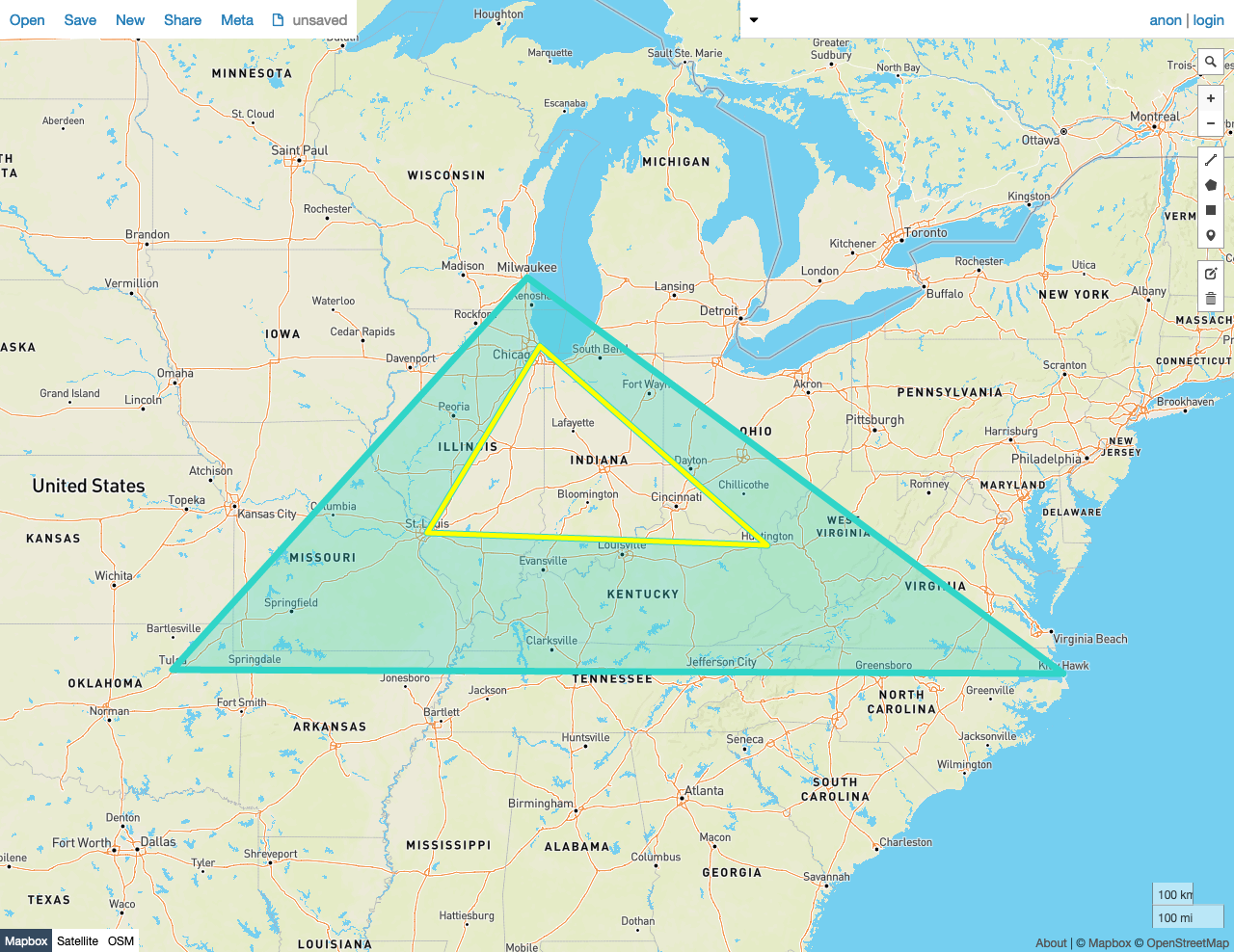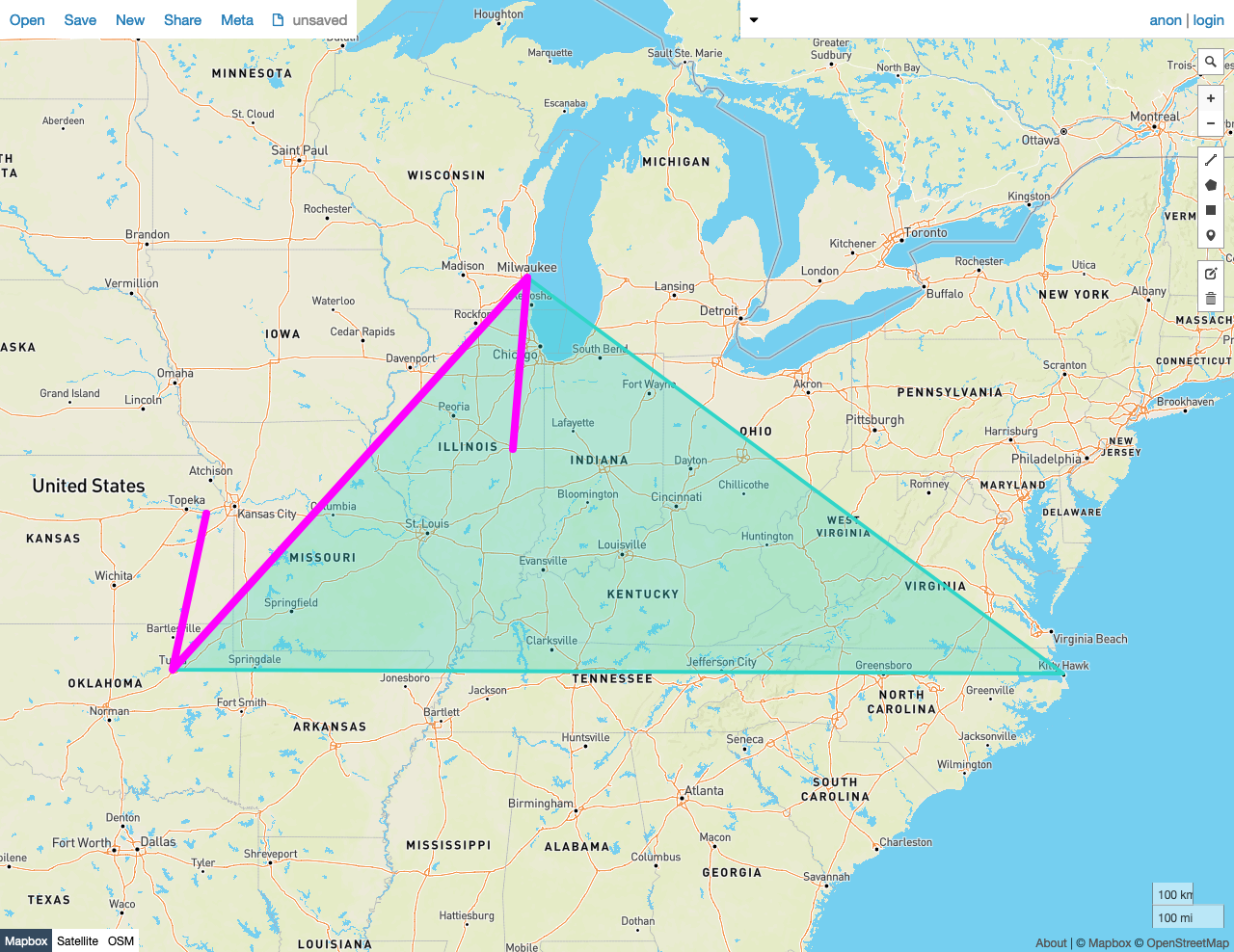On this pageGiven two shapes A and B, `ST_Touches(A, B)` returns `true` if both the following are true:

• At least one point in the set of points that comprises A is also a member of the set of points that make up B.
• No points that make up the interior of A are also part of the interior of B.

In other words, A and B have a point along their boundaries in common (i.e., they "touch"), but none of their interior points intersect. This distinction between shapes touching along a boundary vs. intersecting is also made by the DE-9IM standard.

`ST_Touches` works on the following data types:

Note:

`ST_Touches` will attempt to use any available spatial index to speed up its operation. Use the prefixed variant `_ST_Touches` if you do not want any spatial indexes to be used.

## Examples

Note:

The screenshots in these examples were generated using geojson.io, but they are designed to showcase the shapes, not the map. Representing `GEOMETRY` data in GeoJSON can lead to unexpected results if using geometries with SRIDs other than 4326 (as shown below).

### True

In this example, `ST_Touches` returns `true` because both of the following are true:

• At least one point in the set of Points that comprise Polygon A is a member of the set of points that make up the LineString B.
• No points from the interior of A are also part of the interior of B.
``````SELECT st_touches(st_geomfromtext('SRID=4326;POLYGON((-87.906471 43.038902, -95.992775 36.153980, -75.704722 36.076944, -87.906471 43.038902), (-87.623177 41.881832, -90.199402 38.627003, -82.446732 38.413651, -87.623177 41.881832))'), st_geomfromtext('SRID=4326;LINESTRING(-87.623177 41.881832, -90.199402 38.627003, -82.446732 38.413651, -87.623177 41.881832)'));
``````
``````   st_touches
---------------
true

(1 row)
``````### False

In this example, `ST_Touches` returns `false` because:

• Some points from the interior of the LineString B are also part of the interior of the Polygon A.
``````SELECT st_touches(st_geomfromtext('SRID=4326;POLYGON((-87.906471 43.038902, -95.992775 36.153980, -75.704722 36.076944, -87.906471 43.038902))'), st_geomfromtext('SRID=4326;LINESTRING(-88.243385 40.116421, -87.906471 43.038902, -95.992775 36.153980, -95.235278 38.971667)'));
``````
``````   st_touches
---------------
false
(1 row)
``````